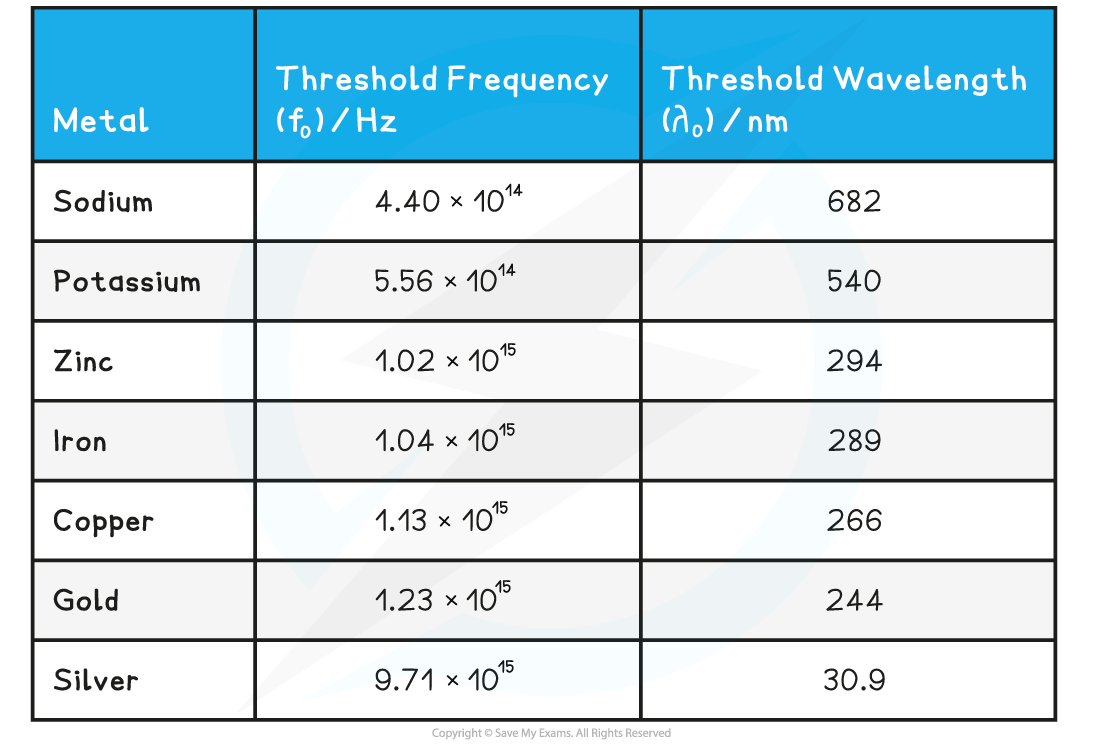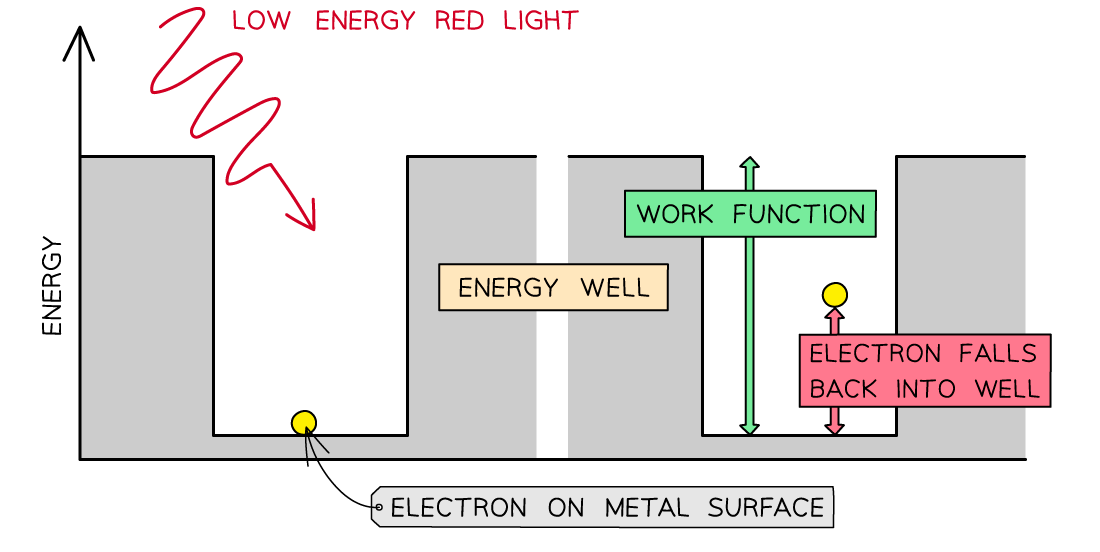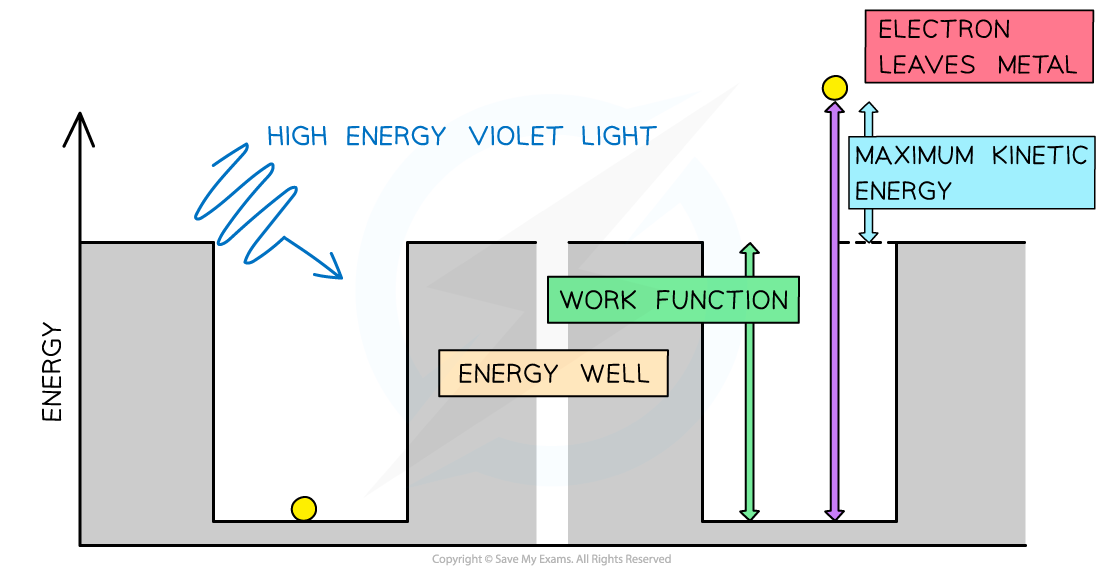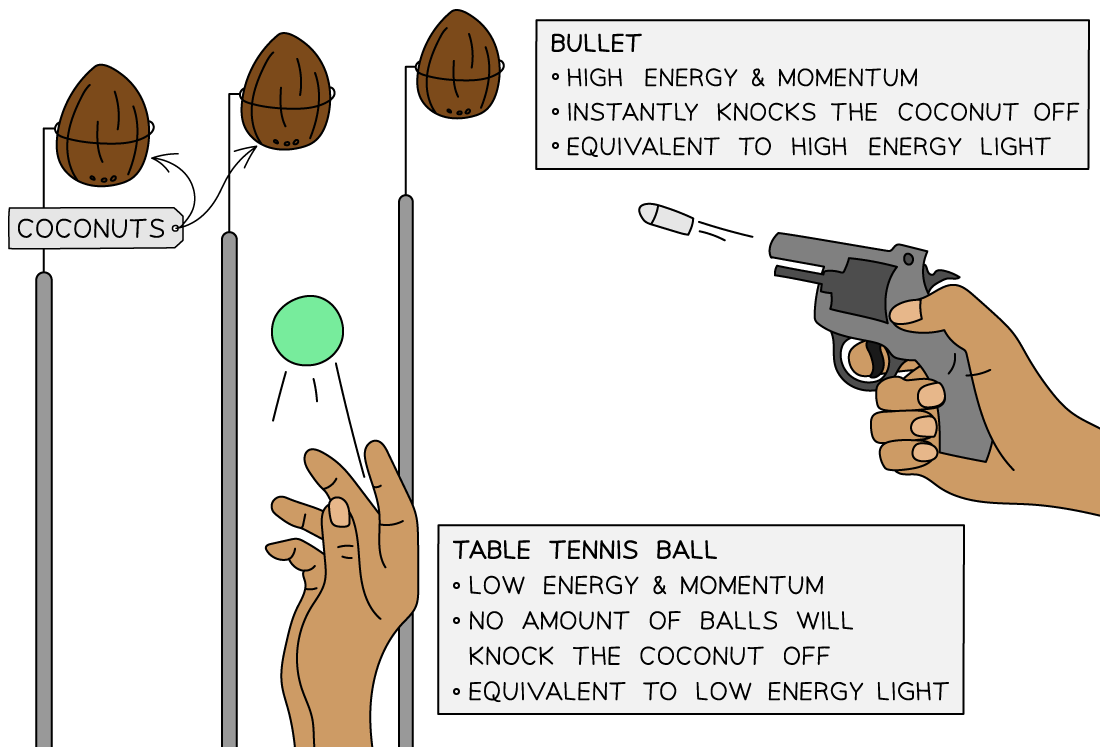# IB DP Physics: HL复习笔记12.1.2 The Photoelectric Effect

### The Photoelectric Effect

• The photoelectric effect is the phenomena in which electrons are emitted from the surface of a metal upon the absorption of electromagnetic radiation
• Electrons removed from a metal in this manner are known as photoelectrons
• The photoelectric effect provides important evidence that light is quantised, or carried in discrete packets
• This is shown by the fact each electron can absorb only a single photon
• This means only the frequencies of light above a threshold frequency will emit a photoelectronPhotoelectrons are emitted from the surface of metal when light shines onto it

#### Threshold Frequency & Wavelength

• The threshold frequency is defined as:

The minimum frequency of incident electromagnetic radiation required to remove a photoelectron from the surface of a metal

• The threshold wavelength, related to threshold frequency by the wave equation, is defined as:

The longest wavelength of incident electromagnetic radiation that would remove a photoelectron from the surface of a metal

• The frequency and wavelength are related by the equation• Since photons are particles of light, v = c (speed of light)
• Threshold frequency and wavelength are properties of a material and vary from metal to metal

Threshold frequencies and wavelengths for different metals#### The Work Function

• The work function Φ, or threshold energy, of a material, is defined as:

The minimum energy required to release a photoelectron from the surface of a metal

• Consider the electrons in a metal as trapped inside an ‘energy well’ where the energy between the surface and the top of the well is equal to the work function Φ
• A single electron absorbs one photon
• Therefore, an electron can only escape from the surface of the metal if it absorbs a photon which has an energy equal to Φ or higherIn the photoelectric effect, a single photon may cause a surface electron to be released if it has sufficient energy

• Different metals have different threshold frequencies and hence different work functions
• Using the well analogy:
• A more tightly bound electron requires more energy to reach the top of the well
• A less tightly bound electron requires less energy to reach the top of the well
• Alkali metals, such as sodium and potassium, have threshold frequencies in the visible light region
• This is because the attractive forces between the surface electrons and positive metal ions are relatively weak
• Transition metals, such as zinc and iron, have threshold frequencies in the ultraviolet region
• This is because the attractive forces between the surface electrons and positive metal ions are much stronger

#### Exam Tip

A useful analogy for threshold frequency is a fairground coconut shy:

• One person is throwing table tennis balls at the coconuts, and another person has a pistol
• No matter how many of the table tennis balls are thrown at the coconut it will still stay firmly in place – this represents the low frequency quanta
• However, a single shot from the pistol will knock off the coconut immediately – this represents the high frequency quanta